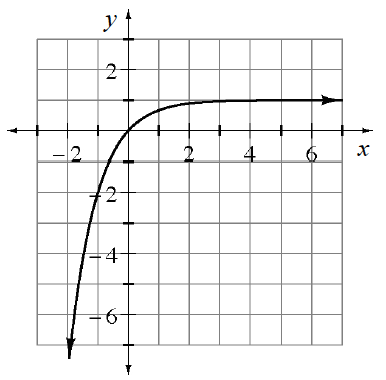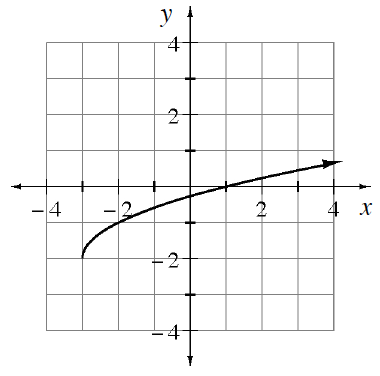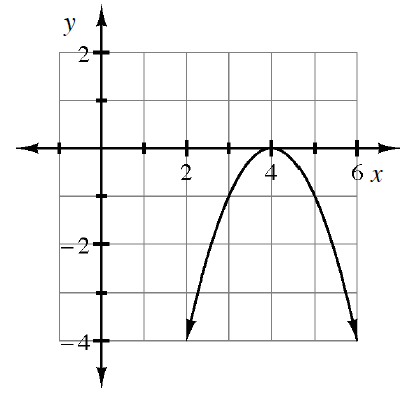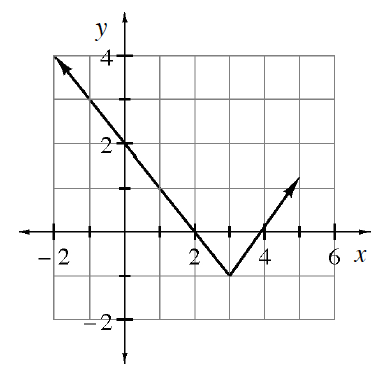### Home > INT1 > Chapter 3 > Lesson 3.1.2 > Problem3-22

3-22.

Determine the domain and range of each of the following graphs.

1.Are there any restrictions on what $x$-values get used in this graph?
Are there any restrictions on the $y$-values that get used in this graph?

Domain: All real numbers.
Range: $y\le1$

1.The arrow indicates the graph continues in only one direction.

Domain: $x\ge−3$
Range: $y\ge−2$

1.Domain: All real numbers.
Range: $y\le0$

1.Domain: All real numbers.
Range: $y\ge−1$